3.3 Density Functional Theory

3.3.1 Introduction

In material science, numerous research topics are related to microscopic processes. The description of these processes often relies on quantities which are not assessable by experiments but can be extracted from atomistic simulations. In the past, so-called first-principles calculations have been successfully employed for the determination of those quantities. These calculations solve the Schrödinger equation of the electrons for a given atomic configuration, and therefore, they do not depend on any fitting parameters. At this point it is should be noted that the knowledge about the exact atomic configuration is often vague, which can sometimes be an serious issue. For instance, it is frequently debated whether the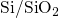interface is abrupt or graded or even has defects, such as suboxides or protrusions. One prominent example of first principle calculations is the Hartree-Fock method. It takes the fundamental exchange interactions2 into account but suffers from a complete neglect of electron correlations and thus fails to reproduce some fundamental properties in solid state physics. Since Hartree-Fock simulations scale badly with the number of electrons, they perform unsatisfactorily with respect to the computational costs when more than only a few tens of atoms are considered. Therefore an alternative approach based on the electron density has been pursued. It is termed density functional theory (DFT)  and can be considered as the workhorse in the field of microscopic simulations. In the following, the basics of this method will be explained.

3.3.2 The Basic Concepts of DFT

The first main idea of DFT is to reformulate the energy of an atomic system as a functional of the ground state electron density instead of the electron wavefunction. The proof of existence for such functionals relies on a one-to-one correspondence between the external potential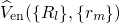and the ground state electron density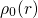. The mapping of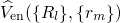ontois obvious: Any Hamiltonian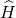with a given external potential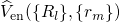has a ground state solution with an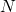-electron wavefunctions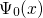, which can be uniquely identified with an electron densityusing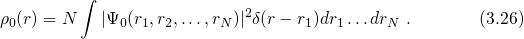The other direction of this mapping (see Fig. 3.3) was proven by the Hohenberg-Kohn (HK) theorem . Due to the resulting one-to-one correspondence between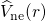and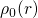, the energy of the atomic system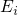can be expressed as a functional of the electron density. Note that DFT is actually restricted to so-called ‘V-representable’ electron densities, however, this is not an issue in the practical use.Figure 3.3: Left: The mapping of the external potential onto the electron density obviously holds true, however, its reverse direction is the central issue of the HK theorem. Right: Electron densities that do not correspond to any external potential violate the requirement of V-representability and thus pose in principle a problem to DFT.

The many-electron wavefunction used in equation (3.26) readswhere its form depends on the ‘combination’ of all spatial electron coordinates. Unfortunately, such an approach would by far exceed any computational capabilities. However, this problem can be overcome using the Kohn-Sham (KS) ansatz, in which the fully-interacting system is replaced by a non-interacting one. This ansatz corresponds to a mean-field approach, where the wavefunction is decomposed into a product of single-electron orbitals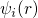. This simplification leads to a neglect of an energy contribution termed ‘correlations’. As a correction, the functional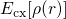must be introduced as an additional term in the Hamiltonian. It is noted that this term does not only account for the correlations but also for the unconsidered exchange interactions. Applying the variation principle to the modified Hamiltonian yields a single-particle Schrödinger equation, also referred to as Kohn-Sham equation in DFT. This equation includes an effective potential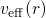, which is produced by the Coulomb forces of all other electrons and nuclei and incorporates the exchange and correlation interactions.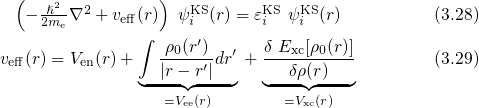The Kohn-Sham orbitalsonly reproduce the correct electron density but actually have no physical meaning. The total energy of the atomic system reads3:Up to now, DFT has been presented as a formally exact framework, however, the complicated part of physics, namely the exchange and correlation interactions, is incorporated in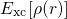. The above eigen-value problem is solved using an iterative method that makes up the computationally expensive step of the DFT calculations. A schematic representation of a self-consistent loop in this numerical method is depicted in Fig. 3.4. With the forces at hands, the energy of a configuration can be minimized with respected to the atomic coordinates. Mathematically, this corresponds to finding the stationary point of a function whose exact form is generally unknown. This task is solved employing iterative methods , such as quasi-Newton methods or the conjugate gradient method for instance. The obtained energy minima corresponds to the stable configurations, which are physically realized and thus important for the determination of stable defect configurations.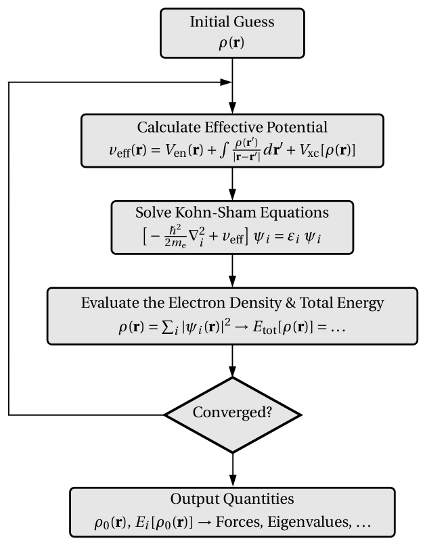Figure 3.4: A flow chart of the iteration scheme. At first, an initial guess for the electron density is assumed, which is required for the calculation of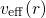, the diagonalization of the Kohn-Sham equations, and the subsequent evaluation of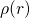along with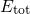. As long as the convergence criterion is not fulfilled, the numerical procedure is continued with the lastinstead of the initial guess. When the criterion is satisfied, various output quantities are computed.

When self-consistency is achieved for this loop, the electronic part of the system is solved. However, the nuclear part described by the Schrödinger equation (2.17) has not been addressed so far. Due to the relatively high nuclei mass, quantum mechanical considerations can be neglected so that the Schrödinger equation (2.17) can be replaced by Newton’s law of motion. The required forces are evaluated according to the Hellmann-Feynman theorem :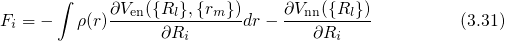3.3.3 Simulation Details

The simulations in this thesis are performed using the Vienna Ab-initio Simulation Package (VASP) , which is based on a plane-wave implementation of DFT. The computational details, especially those important for defect calculations, will be discussed in the following.

The correct description of the exchange-correlation functional takes a crucial role in DFT. The local-density approximation has already achieved satisfactory results for systems with a slowly varying electron density, such as metals . However, it has a tendency termed overbinding, which overestimate binding energies and thus for instance predicts too strong hydrogen bonds with too short bonds lengths. The generalized gradient approximation is a systematic expansion, gives good results in most cases, and corrects for the overbinding . Recently, hybrid functionals  have emerged, which achieve an improved accuracy, especially for the bandgap . However, their use for large-scale investigations of atomic systems is time-demanding. Therefore, the functional based on the parametrization of Perdew, Burke, and Ernzerhof  and provided by the VASP code has been regarded as a reasonable trade-off between accuracy and computation time.

A realistic defect model must contain some of the surrounding atoms of its host material since the atoms of a real defect are connected to the surrounding atomic network and thus are not allowed to move around freely. In order to account for this, the long-range structural relaxations are handled using periodic supercells containing 108 atoms. The host structures have been produced using empirical potential molecular dynamics presented in Section 3.4. The resulting structures were optimized on DFT level employing a conjugate gradient algorithm that minimizes the force on each atom below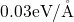. In a further step, the defects were manually introduced by the addition or removal of single atoms, followed by a subsequent structural optimization. Due to the imposed periodic boundary conditions in supercells, the Kohn-Sham orbitals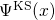were represented by an expansion of plane waves up to a cut-off energy of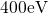. Since the large size of the supercells () decreases the corresponding Brillouin zones, the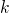-point sampling was restricted to the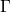-point only.

VASP employs a sophisticated transformation of the Kohn-Sham equations based on the projector-augmented wave (PAW) method , which smoothens the effective potentialnear the cores in order to spare the computationally expensive inclusion of the highly oscillating wavefunctions. The PAW method is one of the most powerful approaches which combines a good transferability to different atomistic configurations and chemical compositions with the required accuracy .

Defect levels for charge capture or emission are calculated by means of the formation energies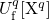, which are defined for a certain charge state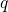and a certain atomic configuration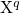of the defect as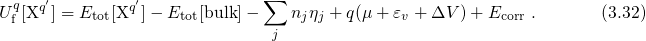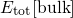stands for the total energy of a supercell containing pure bulk material while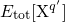the supercell also contains the defect. The third term in equation (3.32) corrects for the different numbers of atoms in both supercells.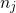gives the number of added (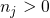) or removed (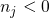) atoms which are required to create the defect from a perfect bulk structure. The subscript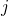refers to the atom type and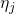denotes the corresponding energy in an atomic reservoir, which must be specified for each individual use case. The fourth term in equation (3.32) accounts for the charge stateof the defect.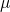is defined as the electron chemical potential referenced with respect to the valence band edgein a bulk-like region.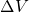corrects the shift in the reference level between two differently charged supercells and is obtained from the difference in the electrostatic potential far distant from the defect. Due to the periodic boundary conditions, charge neutrality must be ensured within a supercell. Thus a homogeneous compensating background charge must be introduced in calculations of charged defects. Its artificial Coulomb interactions are corrected by the term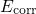.

In DFT literature, one distinguishes between switching and thermodynamic transition levels. The former pertain to a charge capture or emission process, during which the atomic configuration is preserved, and thus they have the same meaning as the electron capture levels and hole emission levels presented in Section 2.3. In DFT they are defined as the difference of formation energies  and can be written as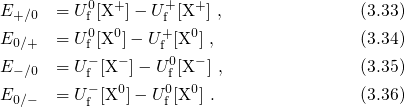Analogously to Section 2.3, energy levels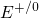and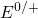apply to a process which neutralizes a positive defect or introduces a positive charge into a neutral defect, respectively. An alternative possibility for the evaluation of the switching transition levels is provided by the Slater-Janak theorem .

The thermodynamic transition levels correspond to the difference between two energy minima, which is also the case for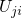in the NMP theory (see section 2.4). In contrast to the switching transition levels, the relaxed configurations for each charge state must be used.